Technical Article

# The Effect of Symmetry on the Fourier Coefficients

May 22, 2022 by Dr. Steve Arar

## Learn about how symmetry in signals can simplify the calculation of the Fourier coefficients when used to find the steady-state response of a circuit.

Finding the Fourier series coefficients of a waveform often involves some relatively tedious calculations. Only by visual inspection of the waveform and without performing a single calculation, it is sometimes possible to determine which coefficients are going to work out to zero. This can be done when the waveform possesses certain types of symmetries. Read on to learn about these symmetries and how they can be used to simplify the calculation of the Fourier coefficients.

### Understanding Fourier Series Coefficients

The following equation can be used to express a periodic signal, f(t), with period T in terms of its Fourier series coefficients:

$$f(t)=a_0 + \sum_{n=1}^{\infty}a_{n}cos(n\omega_{0}t)+\sum_{n=1}^{\infty}b_{n}sin(n\omega_{0}t)$$

Where a0, an, and bn are the Fourier coefficients of the signal; and $$\omega_{0}={\frac{2π}{T}}$$ represents the fundamental frequency of the periodic signal. The frequency nω0 is known as the n-th harmonic of the waveform.

The coefficients can be calculated by the following equations:

$$a_0 = \frac{1}{T}\int_{-\frac{T}{2}}^{+\frac{T}{2}}f(t)dt$$

##### Equation 1.

$$a_n = \frac{2}{T}\int_{-\frac{T}{2}}^{+\frac{T}{2}}f(t)cos(n \omega_0 t)dt$$

##### Equation 2.

$$b_n = \frac{2}{T}\int_{-\frac{T}{2}}^{+\frac{T}{2}}f(t)sin(n \omega_0 t)dt$$

##### Equation 3.

Let’s see how different types of symmetries can simplify the calculation of the above coefficients.

### Even-function Symmetry

Figure 1 shows an example waveform with period T that has even symmetry.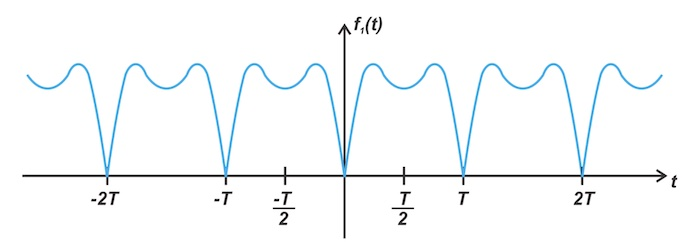##### Figure 1. An example symmetrical waveform with period T.

The graph of an even function is symmetric about the vertical axis (y-axis). In mathematical language, f(t) is even if it satisfies the following condition for all t:

f(–t) = f(t)

A familiar example of even functions is f(t) = cos(t) as it produces the same value for both positive and negative values of a given t. Figure 2 plots f2(t) = cos(2πt).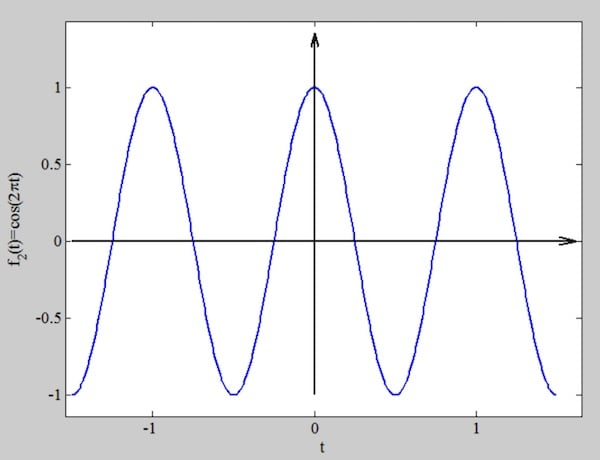##### Figure 2. Example function plot for f2(t) = cos(2πt).

Another even signal is shown in Figure 3.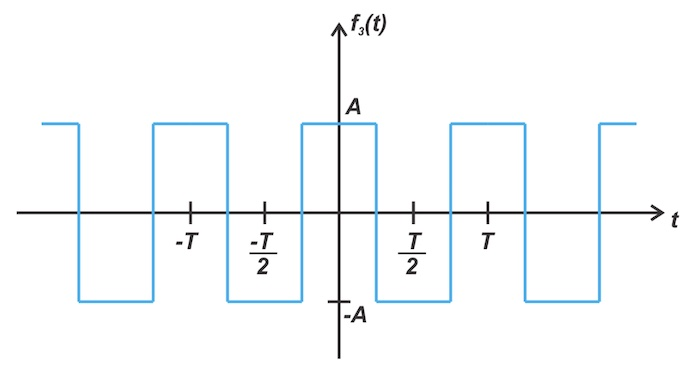##### Figure 3. An example of an even signal.

Although both f1(t) and f3(t) in Figures 1 and 3 have even symmetry, we’ll discuss shortly that f3(t) possesses other levels of symmetry, namely half-wave and quarter-wave symmetries. For now, just notice that the values f3(t) takes over the second half of its period, say the T/2 to T interval, is equal to the negative of the values the signal takes over its previous half-cycle (the 0 to T/2 interval).

### Odd-function Symmetry

An example function with odd symmetry is shown in Figure 4.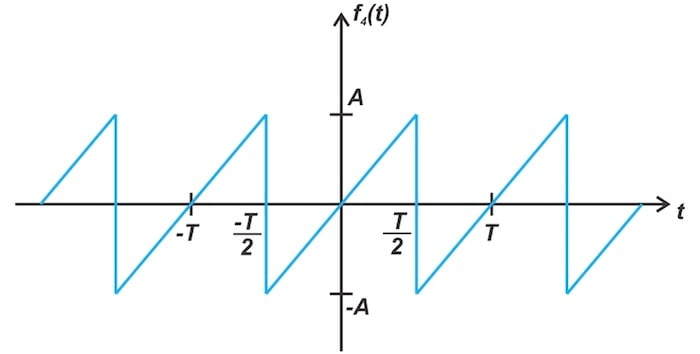##### Figure 4. An example function with odd symmetry.

The graph of an odd function is symmetric about the origin. If f(t) is an odd function, it satisfies the following condition:

f(–t) = –f(t)

Unlike cosine, the sine function is an odd function. Figure 5 shows the plot of f(t) = sin(2πt).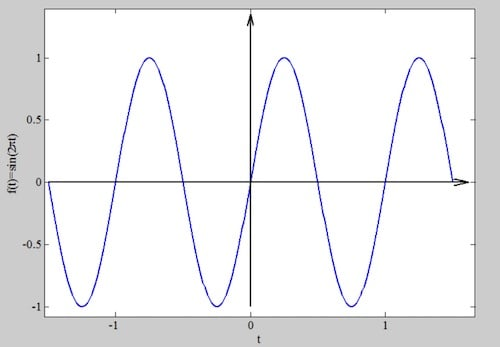##### Figure 5. Example graph plot function for f(t) = sin(2πt).

Another example is depicted in Figure 6.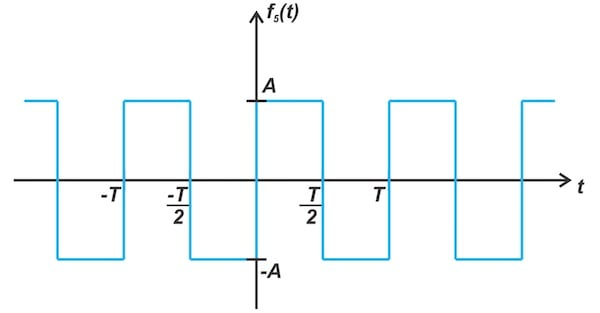##### Figure 6. Another graph example of the same plot.

While both f4(t) and f5(t), depicted in Figures 4 and 6, have odd symmetry, f5(t) possesses half-wave and quarter-wave symmetries as well. Note that the values f5(t) produces over the second half of its period, say the T/2 to T interval, is equal to the negative of the values the signal takes over its previous half-cycle (the 0 to T/2 interval).

Before discussing the effect of these symmetries on Fourier coefficients, let’s take a look at how calculating the integral of a signal can be affected by even and odd types of symmetries.

### Integrating Even and Odd Signals

Consider integrating an even function, f(t), over a symmetric interval from -a to +a. We can write this integral as the sum of two integrals from -a to 0 and 0 to +a, as given below:

$$\int_{-a}^{+a} f(t)dt = \int_{-a}^{0} f(t)dt + \int_{0}^{+a} f(t)dt$$

##### Equation 4.

Previously, we saw that an even function is symmetric about the y-axis. Due to this symmetry, the area under f(t) over the -a to 0 interval is the same as the area over the 0 to +a interval.

Therefore, the above integral can be rewritten as:

$$\int_{-a}^{+a} f(t)dt = 2 \int_{0}^{+a} f(t)dt$$

##### Equation 5.

On the other hand, if we assume that f(t) has odd symmetry, the integral in Equation 4 works out to be zero because the area obtained for the -a to 0 intervals is the negative of that obtained for the 0 to +a interval. This means that assuming odd symmetry, we get:

$$\int_{-a}^{+a} f(t)dt = 0$$

### Effect of Even Symmetry on Fourier Series

If f(t) has even symmetry, we can simplify Equation 1 as follows:

$$a_0 = \frac{1}{T}\int_{-\frac{T}{2}}^{+\frac{T}{2}}f(t)dt=\frac{2}{T}\int_{0}^{+\frac{T}{2}}f(t)dt$$

Moreover, if f(t) is even, you can easily verify that f(t)cos(nω0t) and f(t)sin(nω0t) have even and odd symmetries, respectively.

Therefore,

$$a_n = \frac{2}{T}\int_{-\frac{T}{2}}^{+\frac{T}{2}}f(t)cos(n \omega_0 t)dt = \frac{4}{T}\int_{0}^{+\frac{T}{2}}f(t)cos(n \omega_0 t)dt$$

and,

$$b_n = \frac{2}{T}\int_{-\frac{T}{2}}^{+\frac{T}{2}}f(t)sin(n \omega_0 t)dt = 0$$

Thus, for an even signal, all the bn coefficients are zero.

### Effect of Odd Symmetry on Fourier Series

If f(t) possesses odd symmetry, it can be shown that f(t)cos(nω0t) and f(t)sin(nω0t) have odd and even symmetries, respectively.

Therefore, Equations 1 to 3 simplifies to:

$$a_0 = 0$$

$$a_n = 0$$

$$b_n = \frac{4}{T}\int_{0}^{+\frac{T}{2}}f(t)sin(n \omega_0 t)dt$$

Therefore, for an odd signal, all the an coefficients are zero.

### Half-wave Symmetry

In a signal with half-wave symmetry, each period can be divided into two half-cycles where the two half-cycles have exactly the same shape but are upside down with respect to each other. In other words, the second half-cycle is the same as the first half-cycle inverted about the horizontal axis (time axis). An example waveform with half-wave symmetry is shown in Figure 7.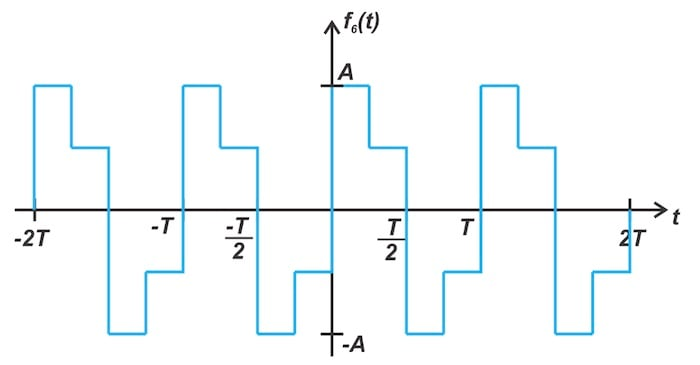##### Figure 7. A half-wave symmetry waveform example.

In mathematical language, this feature can be described by the following condition:

$$f(t) = -f(t-\frac{T}{2})$$

##### Equation 6.

This means that if we shift the signal by T/2 along the time axis and invert it, we’ll obtain the original signal.

With that in mind, how is half-wave symmetry going to affect the Fourier coefficients of the signal?

A signal with half-wave symmetry consists of identical half-cycles with opposite polarities. Due to this alternating feature, we can conclude that the average value of the signal is zero. So half-wave symmetry removes the a0 coefficient right off the bat as this coefficient corresponds to the average value of the signal. However, calculating the other coefficients is a bit complicated.

For an, we start from Equation 2 and divide the integral into two intervals:

$$a_n = \frac{2}{T} \bigg ( \int_{-\frac{T}{2}}^{0}f(t)cos(n \omega_0 t)dt + \int_{0}^{+\frac{T}{2}}f(t)cos(n \omega_0 t)dt \bigg )$$

##### Equation 7.

Now, we'll attempt to rewrite the first integral in terms of the second one in a way that the half-wave symmetry of f(t) can be taken into account.

We can define a new variable as follows:

$$t = τ - \frac{T}{2}$$

For t = –T/2 and t = 0, the new variable is τ = 0 and τ = T/2, respectively.

Also noting that dt = dτ, the first integral can be rewritten as:

$$\int_{-\frac{T}{2}}^{0}f(t)cos(n \omega_0 t)dt = \int_{0}^{\frac{T}{2}}f(\tau - \frac{T}{2})cos\big(n \omega_0 (\tau -\frac{T}{2}) \big)d\tau$$

##### Equation 8.

The cosine term can be simplified as follows:

$$cos\big(n \omega_0 (\tau -\frac{T}{2}) \big) = cos (n \omega_0 \tau -n \pi) = (-1)^{n}cos (n \omega_0 \tau)$$

##### Equation 9.

Applying the half-wave symmetry (Equation 6) and substituting Equation 9, we can express Equation 8 as:

$$\int_{-\frac{T}{2}}^{0}f(t)cos(n \omega_0 t)dt =\int_{0}^{\frac{T}{2}} \big(-f(\tau) \big) (-1)^{n} cos(n \omega_0 \tau) d\tau$$

##### Equation 10.

For an odd n, the above integral is equal to the second integral in Equation 7. However, for an even n, the above integral is equal to the negative of that integral. Therefore, for a signal that has half-wave symmetry, the an coefficients are zero for even values of n. For odd values of n, we have:

$$a_n = \frac{4}{T} \int_{0}^{+\frac{T}{2}}f(t)cos(n \omega_0 t)dt$$

##### Equation 11.

Following a similar procedure, it can be shown that the bn coefficients are also zero for even values of n. For odd values of n, we have:

$$b_n = \frac{4}{T} \int_{0}^{+\frac{T}{2}}f(t)sin(n \omega_0 t)dt$$

### Quarter-wave Symmetry

When a periodic waveform possesses both half-wave symmetry and even/odd type of symmetry, it is said to have quarter-wave symmetry. For example, the waveform in Figure 3 possesses half-wave symmetry as well as even symmetry. On the other hand, the waveform in Figure 6 is an odd signal with half-wave symmetry. Therefore, these two signals are said to have quarter-wave symmetry.

For an even signal with half-wave symmetry, the bn coefficients are zero for all n due to the even symmetry property. Also, the half-wave symmetry makes a0 and the an coefficients for even n vanish. Therefore, for an even signal with half-wave symmetry, only the an coefficients for odd n can be non-zero. These coefficients can be found by applying Equation 11, which is repeated below for convenience:

$$a_n = \frac{4}{T} \int_{0}^{+\frac{T}{2}}f(t)cos(n \omega_0 t)dt$$

With the quarter-wave symmetry, the signal exhibits symmetry about the midpoint of the positive and negative half-cycles. Therefore, the above integral can be simplified to:

$$a_n = \frac{8}{T} \int_{0}^{+\frac{T}{4}}f(t)cos(n \omega_0 t)dt$$

Similarly, if a function is odd and has half-wave symmetry, the an coefficients are zero due to the odd symmetry property. Again, a0 is zero because a half-wave signal has an average value of zero. Due to the half-wave symmetry of the signal, only the bn coefficients for odd n can be non-zero. For odd n, bn is given by:

$$b_n = \frac{4}{T} \int_{0}^{+\frac{T}{2}}f(t)sin(n \omega_0 t)dt$$

Which again can be simplified to:

$$b_n = \frac{8}{T} \int_{0}^{+\frac{T}{4}}f(t)sin(n \omega_0 t)dt$$

### Examining Two Common Waveforms

Consider the sawtooth waveform shown in Figure 8.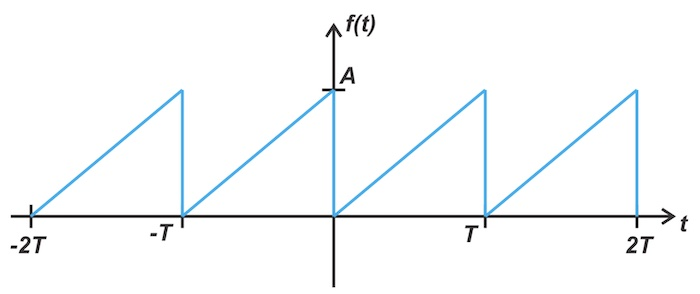##### Figure 8. An example of a sawtooth waveform.

Using Equations 1 to 3, the above waveform can be expressed as follows:

$$f(t)=\frac{A}{2}-\frac{A}{\pi}\sum_{n=1}^{\infty}\frac{sin(n \omega_{0}t)}{n}$$

##### Equation 13.

To more easily relate the waveform to its Fourier coefficients, let’s subtract the DC value from the signal, which gives us the waveform in Figure 9.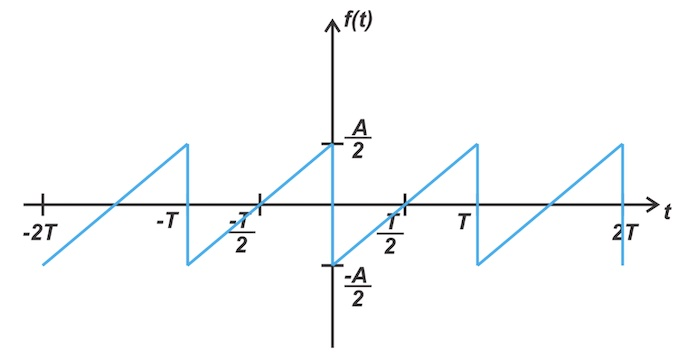##### Figure 9. Example odd function waveform where the DC value is subtracted from the signal.

Eliminating the DC value, we obtain an odd function. Therefore, we can conclude that only the bn coefficients can be non-zero, which is consistent with Equation 13. As the final example, we’ll look at the triangle wave shown in Figure 10.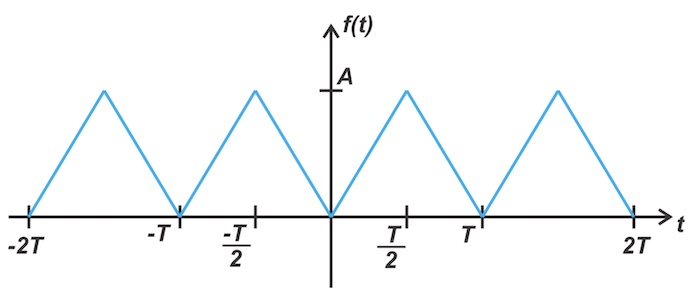##### Figure 10. An example triangle wave.

The Fourier series representation of the function is as follows:

$$f(t)=\frac{A}{2}-\frac{4A}{\pi^{2}}\sum_{n=1}^{\infty}\frac{cos \big((2n-1) \omega_{0}t \big)}{(2n-1)^2}$$

##### Equation 14.

Again, by subtracting the average value, we can obtain the waveform in Figure 11.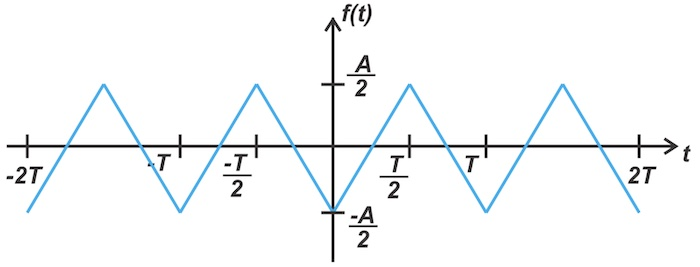##### Figure 11. A half-wave symmetrical waveform.

In this case, we have an even signal that exhibits half-wave symmetry. Therefore, we should have b= 0 for all n; and a= 0 for even n. This is consistent with Equation 14.

In this article, we explored the effect of different types of symmetries on the Fourier coefficients. Armed with this knowledge, you should now be able to predict which coefficients are going to turn out to be zero when the signal possesses a certain type of symmetry.